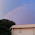Tuesday, July 12, 2016

Universality in mathematics

Physicists tend to think of universality (the details don't matter)  as a physical phenomena. However, in the early days of this blog I posted about how I became aware that the central limit theorem in mathematics is an example of universality.

Terence Tao has a nice article intended for a popular audience ,“E pluribus unum: from complexity, universality” that gives several examples of universality in mathematics and physics. The figures are particularly nice.
Some of the examples given include the law of large numbers, the central limit theorem, and Benford's law.

"A histogram of the first two digits of accounts payable data of a major software company, together with the Benford's law prediction. (Source: Journal of Accountancy)"

The nicest part of the article is the discussion of the connections between random matrix theory, energy level spacings in nuclear physics, and the spacing of prime numbers and zeros of the Riemann zeta function. Universality is reflected in them all being described by the same probability distribution.

"A histogram of spacings between bus arrival times in Cuernavaca (Mexico), in crosses; the solid line is the prediction from random matrix theory. (Source: Krbalek-Seba, J. Phys. A., 2000)"

"Spacing distribution for a billion zeroes of the Riemann zeta function, and the corresponding prediction from random matrix theory. (Source: Andrew Odlyzko, Contemp. Math. 2001)"

These connections between mathematics and physics raise subtle philosophical questions as to whether the emergence of universality in physics is "just" a reflection of universality in mathematics.

1.Yes, the renormalization group and the central limit theorem are closely related. A version of the central limit theorem can be arrived at by RG-like methods: http://web.math.princeton.edu/facultypapers/Sinai/KolmogorovLec07.pdf

Similarly, universal phenomena in chaos such as the Feigenbaum number can be deduced by RG-like methods:
https://arxiv.org/abs/1210.2262

2.Do you know of a pdf version of Tao's piece that's not behind a paywall?

1.No. Sorry. Pity.